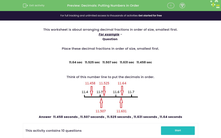# Decimals: Putting Numbers in Order

In this worksheet, students arrange decimal fractions in order of size, smallest first. A number line is used to illustrate the example.Key stage:  KS 2

Curriculum topic:   Number: Fractions, Decimals and Percentages

Curriculum subtopic:   Use up to Three Decimal Places

Difficulty level:#### Worksheet Overview

This worksheet is about arranging decimal fractions in order of size, smallest first.

For example -

Question

Place these decimal fractions in order of size, smallest first.

11.64 sec 11.525 sec 11.507 sec 11.631 sec 11.458 sec

Think of this number line to put the decimals in order.Answer  11.458 seconds , 11.507 seconds , 11.525 seconds , 11.631 seconds , 11.64 seconds

Look at the digits in the columns from left to right. The numbers above all start with 11, so then look at the tenths column.

If the numbers have the same digit in the tenths column, move on to the next column to the right - the hundredths.

### What is EdPlace?

We're your National Curriculum aligned online education content provider helping each child succeed in English, maths and science from year 1 to GCSE. With an EdPlace account you’ll be able to track and measure progress, helping each child achieve their best. We build confidence and attainment by personalising each child’s learning at a level that suits them.

Get started